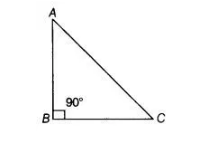# An isosceles right triangle has area 8 cm2.`
Question:

An isosceles right triangle has area 8 cm2. The length of its hypotenuse is

(a) √32 cm

(b) √16 cm

(c) √48 cm

(d) √24

Solution:

(a) Given, area of an isosceles right triangle $=8 \mathrm{~cm}^{2}$ Area of an isosceles triangle $=1 / 2$ (Base $x$ Height) $\Rightarrow 8=1 / 2$ (Base $\times$ Base $)$

$[\therefore$ base $=$ height, as triangle is an isosceles triangle $]$ $\Rightarrow(\text { Base })^{2}=16 \Rightarrow$ Base $=4 \mathrm{~cm}$In ΔABC, using Pythagoras theore

AC2 = AB2 + BC2 = 42 + 42 = 16 + 16

=> AC2 = 32 => AC = √32 cm
[taking positive square root because length is always positive]

Hence, the length of its hypotenuse is √32 cm.